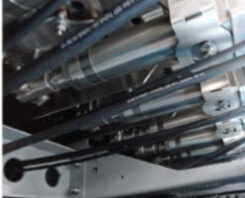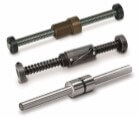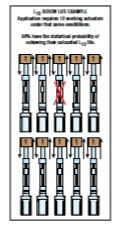# Electric actuator life in units of time: ball & roller screw actuators

By Tolomatic on August 9, 2016A machine’s useful life depends on the life of its critical components. And machine designers  frequently hear the question, “How long can I expect this machine to keep working?”

When electric linear actuators are used, calculating life can be straight-forward for ball screw and roller screw actuators.  Since these screw types incorporate rolling elements as essential parts, you can use the L10 life formula for ball bearings.

For a thorough explanation of how to use the L10 life formula when estimating electric actuator screw life, see our newest guide, Actuator Life: How to estimate for ball and roller screw actuators. Get your copy here.

## Distance versus time

The result of an L10 life calculation is in inches or millimeters of travel. This is useful information; however, the individuals asking you how long your machine will last probably want the information in units of time, like days, months or years.

(Of course, L10 is a theoretical calculation of life based on load. As a seasoned designer, you’ll also consider the many other factors that can affect actuator life like the environment and alignment.)

This blog will show you how to convert an L10 result to units of time. But first a quick review.

##Important terms

Dynamic Load Rating (DLR): Provided by the manufacturer and represented by the letter C. DLR is a constant load under which a ball bearing device will achieve 1 million revolutions (rotations).

Equivalent Dynamic Load:  Must be calculated when an application has a varying load. It’s a dynamic load which, if applied constantly, would have the same effect on screw life as the combined actual loads. Represented by the symbol, Pe.

L10 (or B10) Life: L10 life for a group of identical screw actuators operating under the same conditions is the number of revolutions which 90% of these actuators have the statistical probability to achieve (or at which 10% can be expected to fail).

Here are the basic formulas.

## L10 life with a constant load

L10 = (C/Pe)3 x l

C = Dynamic load rating (lbf) or (N)

l = Screw lead (in/rev) or (mm/rev)

## L10 life with a varying load

First, calculate the Equivalent Load, Pe:

Pe = 3 √{ [L1(P1)3+L2(P2 )3+L3(P3 )3+Ln(Pn )3]/L}

Pe = Equivalent load (lbs) or (N)

Pn = Each increment at different load (lbs) or (N)

L = Total distance traveled per cycle (extend + retract stroke) (L = L1 + L2 + L3 + Ln)

Ln = Each increment of stroke (in) or (mm) at different load

Then use the calculated Pe in the life calculation formula: L10 = (C/Pe)3 x l

##How to estimate actuator life in time

Here’s what you need to know for this calculation:

L10    life calculation in distance

L         total distance traveled per cycle (extend + retract stroke)

CpM   Number of cycles per minute

HpD    Number of hours operated per day

DpY    Number of days of operation per year

Put that information into one of these formulas:

Life Estimate in Years =

(L10 / L) / [(CpM) X 60 min/hr X (HpD) X (DpY)]

Life Estimate in Days =

(L10 / L) / [(CpM) X 60 min/hr X (HpD)]

This gives you the estimated life of the ball screw or roller screw actuator in units of time.

Now you can begin to answer the question “How long can I expect this machine to keep working?”  In an upcoming blog we’ll consider comparing actuators for an application.